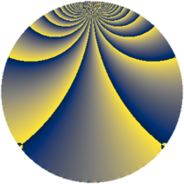Properties

 Label 1568.1.blLevel $1568$ Weight $1$ Character orbit 1568.bl Rep. character $\chi_{1568}(117,\cdot)$ Character field $\Q(\zeta_{24})$ Dimension $8$ Newform subspaces $1$ Sturm bound $224$ Trace bound $0$

Related objects

Defining parameters

 Level: $$N$$ $$=$$ $$1568 = 2^{5} \cdot 7^{2}$$ Weight: $$k$$ $$=$$ $$1$$ Character orbit: $$[\chi]$$ $$=$$ 1568.bl (of order $$24$$ and degree $$8$$) Character conductor: $$\operatorname{cond}(\chi)$$ $$=$$ $$224$$ Character field: $$\Q(\zeta_{24})$$ Newform subspaces: $$1$$ Sturm bound: $$224$$ Trace bound: $$0$$

Dimensions

The following table gives the dimensions of various subspaces of $$M_{1}(1568, [\chi])$$.

Total New Old
Modular forms 72 40 32
Cusp forms 8 8 0
Eisenstein series 64 32 32

The following table gives the dimensions of subspaces with specified projective image type.

$$D_n$$ $$A_4$$ $$S_4$$ $$A_5$$
Dimension 8 0 0 0

Trace form

 $$8 q + O(q^{10})$$ $$8 q + 4 q^{16} + 4 q^{18} + 8 q^{22} + 4 q^{23} - 8 q^{43} - 4 q^{44} + 4 q^{53} - 4 q^{67} - 4 q^{74} - 8 q^{92} + O(q^{100})$$

Decomposition of $$S_{1}^{\mathrm{new}}(1568, [\chi])$$ into newform subspaces

Label Dim. $$A$$ Field Image CM RM Traces $q$-expansion
$a_{2}$ $a_{3}$ $a_{5}$ $a_{7}$
1568.1.bl.a $8$ $0.783$ $$\Q(\zeta_{24})$$ $D_{8}$ $$\Q(\sqrt{-7})$$ None $$0$$ $$0$$ $$0$$ $$0$$ $$q-\zeta_{24}^{5}q^{2}+\zeta_{24}^{10}q^{4}+\zeta_{24}^{3}q^{8}+\cdots$$